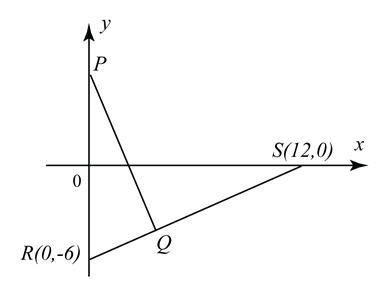# 6.8.1 Coordinate Geometry Long Questions (Question 1 & 2)

Question 1:The diagram shows a straight line PQ which meets a straight line RS at the point Q. The point P lies on the y-axis.
(a) Write down the equation of RS in the intercept form.
(b) Given that 2RQ = QS, find the coordinates of Q.
(c) Given that PQ is perpendicular to RS, find the y-intercept of PQ.

Solution:
(a)
Equation of RS
$\begin{array}{l}\frac{x}{12}+\frac{y}{-6}=1\\ \frac{x}{12}-\frac{y}{6}=1\end{array}$

(b)

(c)

Point Q = (4, –4), m = –2
Using y = mx+ c
–4 = –2 (4) + c
c = 4
y–intercept of PQ = 4

Question 2:The diagram shows a trapezium PQRS. Given the equation of PQ is 2y x – 5 = 0, find
(a) The value of w,
(b) the equation of PS and hence find the coordinates of P.
(c) The locus of M such that triangle QMS is always perpendicular at M.

Solution:
(a)

(b)
$\begin{array}{l}{m}_{PQ}=\frac{1}{2}\\ {m}_{PS}=-\frac{1}{{m}_{PQ}}=-\frac{1}{\frac{1}{2}}=-2\end{array}$

Point S = (4, –3), m = –2
yy1 = m (xx1)
y – (–3) = –2 (x – 4)
y + 3 = –2x + 8
y = –2x + 5
Equation of PS is y = –2x + 5

PS is y = –2x + 5—–(1)
PQ is 2y = x + 5—–(2)
Substitute (1) into (2)
2 (–2x + 5) = x + 5
–4x + 10 = x + 5
–5x = –5
x = 1
From (1), y = –2(1) + 5
y = 3
Coordinates of point P = (1, 3).

(c)

Hence, the equation of locus of the moving point M is
x2 + y2– 9x – 2y + 5 = 0.

### 1 thought on “6.8.1 Coordinate Geometry Long Questions (Question 1 & 2)”

1.helped me to warm up a little bit before exam. thx~~